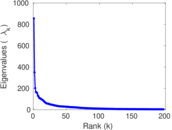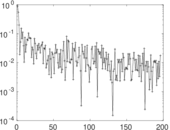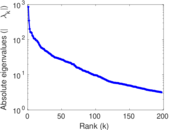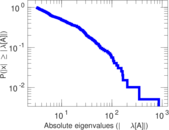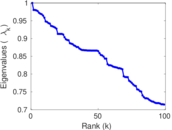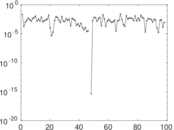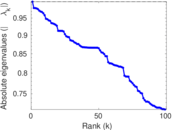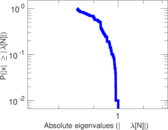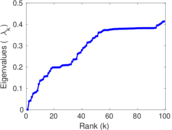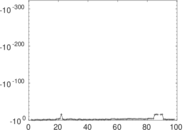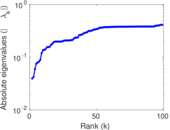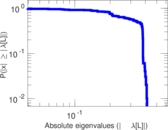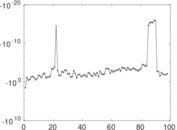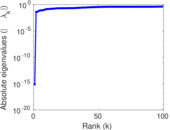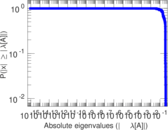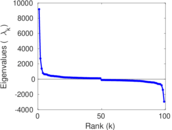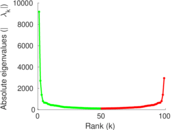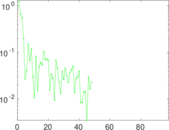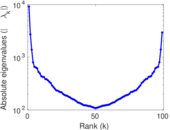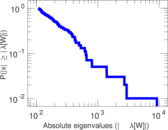# Wikipedia edits (nrm)

This is the bipartite edit network of the Nouormand Wikipedia. It contains users and pages from the Nouormand Wikipedia, connected by edit events. Each edge represents an edit. The dataset includes the timestamp of each edit.

 Code `nrm` Internal name `edit-nrmwiki` Name Wikipedia edits (nrm) Data source http://dumps.wikimedia.org/ AvailabilityDataset is available for download Consistency checkDataset passed all tests Category Authorship network Dataset timestamp 2017-10-20 Node meaning User, article Edge meaning Edit Network formatBipartite, undirected Edge typeUnweighted, multiple edges Temporal dataEdges are annotated with timestamps

## Statistics

 Size n = 8,944 Left size n1 = 891 Right size n2 = 8,053 Volume m = 201,831 Unique edge count m̿ = 83,068 Wedge count s = 79,205,742 Claw count z = 67,563,235,681 Cross count x = 48,105,958,410,143 Square count q = 464,288,828 4-Tour count T4 = 4,031,465,296 Maximum degree dmax = 24,151 Maximum left degree d1max = 24,151 Maximum right degree d2max = 299 Average degree d = 45.132 2 Average left degree d1 = 226.522 Average right degree d2 = 25.062 8 Fill p = 0.011 577 1 Average edge multiplicity m̃ = 2.429 71 Size of LCC N = 8,379 Diameter δ = 12 50-Percentile effective diameter δ0.5 = 3.146 93 90-Percentile effective diameter δ0.9 = 4.981 11 Median distance δM = 4 Mean distance δm = 3.457 90 Gini coefficient G = 0.819 137 Balanced inequality ratio P = 0.192 352 Left balanced inequality ratio P1 = 0.058 667 9 Right balanced inequality ratio P2 = 0.243 382 Relative edge distribution entropy Her = 0.766 947 Power law exponent γ = 1.717 79 Tail power law exponent γt = 3.071 00 Tail power law exponent with p γ3 = 3.071 00 p-value p = 0.000 00 Left tail power law exponent with p γ3,1 = 1.421 00 Left p-value p1 = 0.000 00 Right tail power law exponent with p γ3,2 = 8.911 00 Right p-value p2 = 0.013 000 0 Degree assortativity ρ = −0.107 643 Degree assortativity p-value pρ = 1.543 26 × 10−212 Spectral norm α = 855.769 Algebraic connectivity a = 0.039 474 8 Spectral separation |λ1[A] / λ2[A]| = 2.451 02 Controllability C = 7,193 Relative controllability Cr = 0.811 119

## Plots

### Fruchterman–Reingold graph drawing### Degree distribution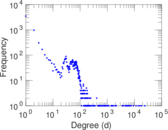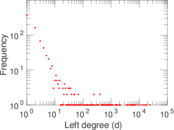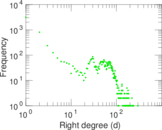### Cumulative degree distribution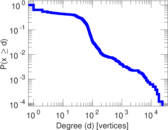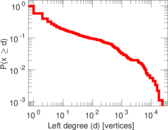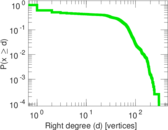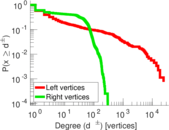### Lorenz curve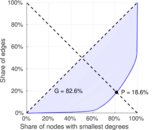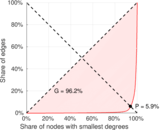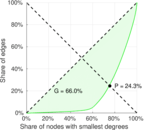### Spectral distribution of the adjacency matrix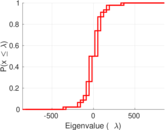### Spectral distribution of the normalized adjacency matrix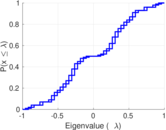### Spectral distribution of the Laplacian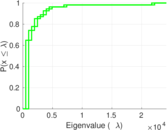### Spectral graph drawing based on the adjacency matrix### Spectral graph drawing based on the Laplacian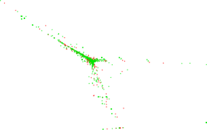### Spectral graph drawing based on the normalized adjacency matrix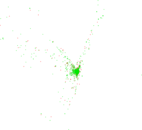### Degree assortativity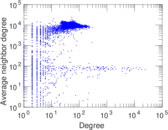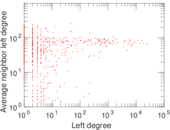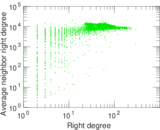### Zipf plot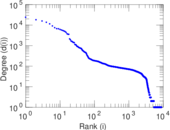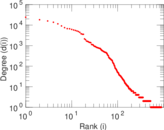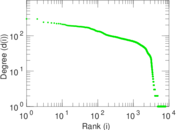### Hop distribution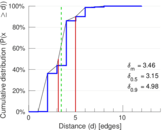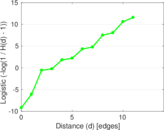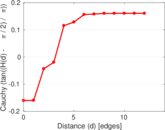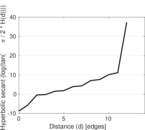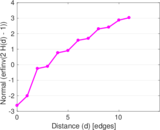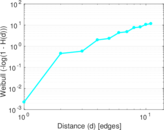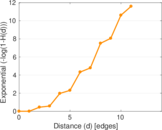### Double Laplacian graph drawing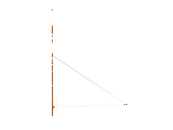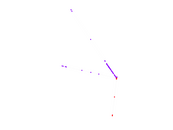### Delaunay graph drawing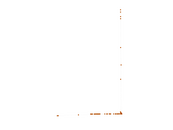### Edge weight/multiplicity distribution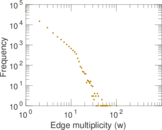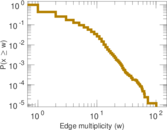### Temporal distribution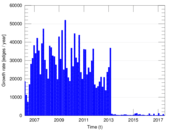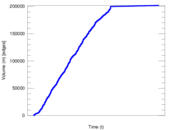### Temporal hop distribution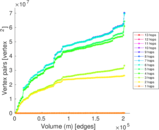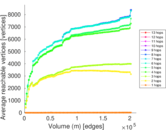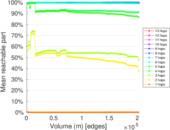### Diameter/density evolution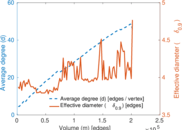### Matrix decompositions plots